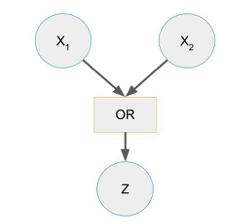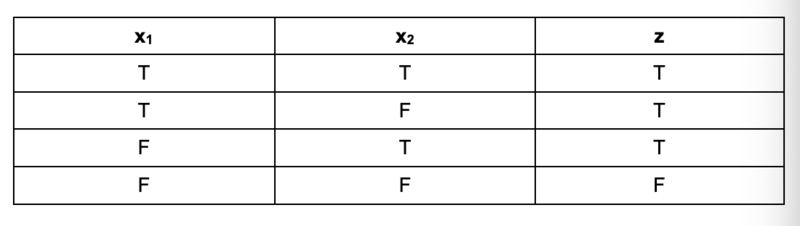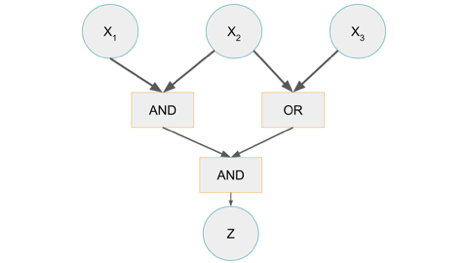# Complexity Explorer Santa Few Institute## Computation in Complex Systems (Spring 2022)

This course is no longer in session.

• Course Introduction
• Two Kinds of Paths
• Polynomials vs. Exponentials
• Divide and Conquer
• Big O and All that
• When the details don't matter
• Exam
• Divide and Conquer Redux
• Dynamic Programming
• Greedy Algorithms
• Reductions and Translations
• Lessons So Far
• The Best of All Possible Algorithms
• Complexity Wrap-Up
• Exam
• Finding versus Checking
• Circuits and Formulas
• More NP-complete Problems
• P versus NP Problem
• Existence and Nonexistence
• Above and Beyond
• Exam
• Real World Problems
• Phase Transitions
• Random Problems
• Solvability Threshold
• Modeling Differential Equations
• Landscapes, Clustering, Freezing, and Hardness
• Exam
• Building Blocks: Recursive Functions
• Building Blocks: Partial Recursive Functions
• λ Calculus
• Turing Machines
• The Halting Problem
• The Grand Unified Theory of Computation
• The Analytical Engine
• Cellular Automata
• Tile-Based Computation
• Dynamical Systems
• Exam
• More from Cris Moore
• Other ComplexityExplorer resources

#### 3.7 Quiz 3 (self-assessment) » Quiz 3: Explanation

Q1. Given the following circuit, what values of x1 and x2 result in z being FALSE?(A) x1 = TRUE, x2 = TRUE

(B) x1 = TRUE, x2 = FALSE

(C) x1 = FALSE, x2 = TRUE

(D) x1 = FALSE, x2 = FALSE

Explanation: This circuit is an OR gate: z is dependent upon the relationship between x1 and x2, defined through the “OR” logical operator. The truth table of this expression is shown below, and the only way for z to be FALSE is for x1 and x2 both to be FALSE as well.Q2. Given the following circuit, what values of x1, x2, and x3 result in z being TRUE?(A) x1 = TRUE, x2 = FALSE, x3 = TRUE.

(B) x1 = FALSE, x2 = TRUE, x3 = TRUE

(C) x1 = TRUE, x2 = TRUE, x3 = FALSE

(D) x1 = FALSE, x2 = FALSE, x3 = TRUE

Explanation: This is easier to explain from the bottom up: for z to be TRUE, the second level (the AND and OR boxes directly below the x values) must both evaluate to TRUE, since they are linked to z via the lower AND box. Since the AND box connected to x1 and x2 must be TRUE, x1 and x2 must both be TRUE. Likewise, since the OR box connected to x2 and x3 must be TRUE, either x2 or x3 must be TRUE. Option (c) is the only option which satisfies these criteria.

Q3. Which description properly describes the difference between a P, and NP problem?

(A) A P problem must be solved through brute force.

(B) NP problems can always be reduced to P.

(C) NP problems are checkable but not solvable in polynomial time.

(D) P problems are “tougher” than all NP problems.

Explanation: A problem is in P (polynomial time) if it can be both verified (checked) and solved in polynomial time. In contrast, NP problems can be verified in polynomial time, but require exponential time to solve. NP problems cannot be reduced to P, given current computational theory, and since they require exponential time to solve - usually requiring brute force - they are considered “tougher” to solve than P problems.

Q4. A solution to Sudoku can be checked in polynomial time, but the exact solution can only be found in exponential time. What class of problems contains Sudoku?

(A) P

(B) NP

(C) Undecidable

Explanation: A problem is NP if it takes exponential time to solve, and if a single solution can be verified in polynomial time. A solution to Sudoku can easily be verified for impossibilities (e.g., the same number in a 3x3 square or on the same line), but in order to find a solution, all solutions must be checked. Therefore, this problem is in NP.

If Sudoku was in P, then it would be both verifiable and solvable in polynomial time, and there would be a way to find a solution without iterating through every solution.

It is worth noting that a human solving sudoku does not iterate through all possible solutions - a partial solution is already given, and we use a variety of heuristics to solve the problem. A computational solution is quite different from human-based algorithms.

Q5. Chess, tic-tac-toe, and many other games can be classified as in the PSPACE class of algorithms. Why is this?

(A) These games are solvable in exponential time with exponential amounts of memory.

(B) They are solvable only using vast amounts of memory.

(C) They are solvable using polynomial time with exponential memory.

(D) They are solvable using exponential time with polynomial memory.

Explanation: From lecture 3.6 (Above & Beyond), PSPACE problems are those which can be solved with polynomial memory. They use a variety of methods, such as binary search trees, to enumerate possible solutions and to eliminate branches which contain contradictions and are therefore impossible. These problems also commonly take exponential time to solve, since all possible solutions must be enumerated in order to prove a solution exists.